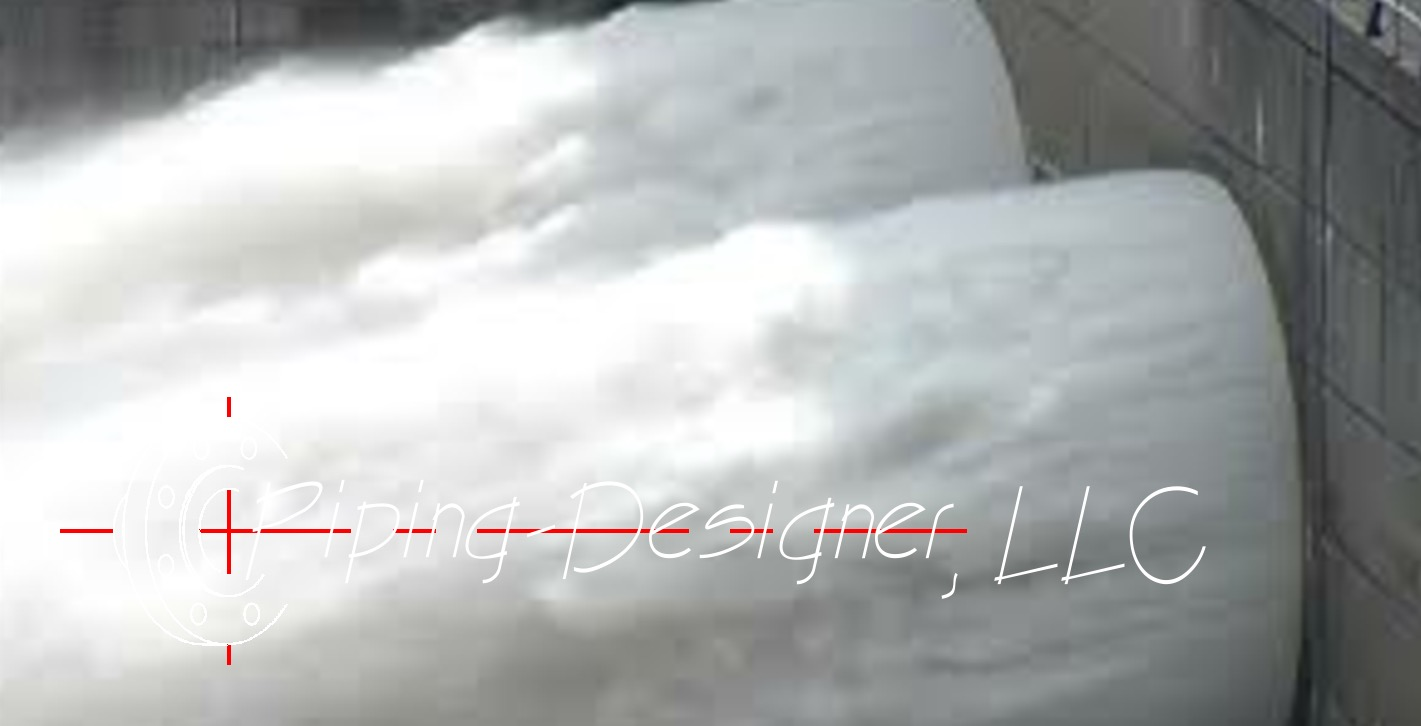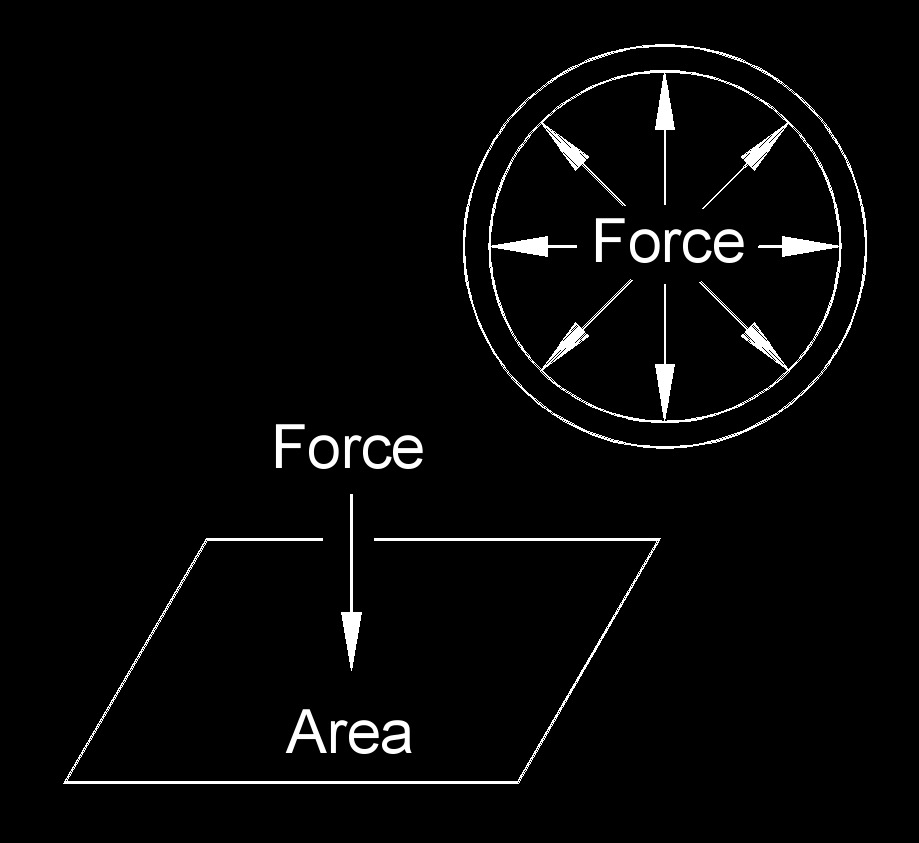# Pressure

Written by Jerry Ratzlaff on . Posted in Thermodynamics

##Pressure, abbreviated as p, is one of the most important concepts in piping design.  Simply put, it is the force exerted perpendicular to the surface of an object and is expressed as force per unit area.  Why is this important?  The purpose of a pipe or pressure vessel is to keep pressure contained in a specific location.  Differential pressure is also what causes fluids to move.  It will always flow from high to low pressure.  Pressure differences introduced by a pump or compressor will cause the fluid to flow.

Pressure is a scalar quantity having direction, some of these include area, density, energy, entropy, length, mass, power, speed, temperature, volume, and work.

There are several different types of pressure, each used for different things.  For example:

Absolute Pressure - Absolute pressure is the pressure in the piping that takes into account the atmospheric pressure.  No matter where you are on earth, the atmosphere exerts a pressure force on everything.  Absolute Pressure is useful when calculating the net positive suction pressure for a pump.

Gauge Pressure - Gauge Pressure is the difference of pressure that is measured by the gauge.

Differential Pressure - Differential pressure is used to describe the amount of pressure created by a pump or compressor.  It also is used for pressure drop in a pipe, piece of equipment or an orifice plate.$$\large{ p = \frac{F}{A} }$$          Where: $$\large{ p }$$ = pressure $$\large{ A }$$ = area $$\large{ F }$$ = force Solve for: $$\large{ A = \frac{F}{p} }$$ $$\large{ F = p \; A }$$

## Typical Units

Some common units for pressure are as follows.  For a more complete list, visit the pressure conversion page

INTERNATIONAL SYSTEM OF UNITS, SI

ENGLISH UNITS

Bar Inches of Mercury
Centimeter of Mercury Inches of Water
Centimeter of Water Kip per Foot2, KSF
Pascals, Pa Kip per Inch2, KSI
Pound per Foot2, PSF
Pound per Inch2, PSI

## Atmospheric Pressure

Atmospheric pressure, abbreviated as $$p_a$$, is the pressure exerted upon the earth's surface by the air because of the gravitational attraction of the earth. Standard atmosphere pressure at sea level is 14.7 pounds per square inch (psi).  Measured with a barometer.

## Negative Pressure

Pressure is normally positive, but negative pressure is when the enclosed pressure is lower than the area around it.

## Pressure Instruments

In piping design, pressure is measured several different ways.  On a Piping & Instrumentation Diagram, the typical instruments are:

Pressure Indicator - A pressure indicator is a pressure gauge.  It is a mechanical device, that is calibrated to display a pressure.

Pressure Transmitter or pressure indicating transmitter - This is used to display the pressure in the equipment and send an analog signal to a computer for futher processing.  It might be used as an alarm in case the pressure gets outside normal operating conditions.

Pressure Switch - A pressure switch is used to send a digital signal (yes or no, 1 or 0) to a computer for an action to be performed.  E.g. send an alarm, turn off a pump, etc.